MC
##### Matteo Casoni4 Messages

•

100 Points

Fri, Nov 6, 2020 7:33 AM

Closed

# Lightroom Classic: crash when export photo or making massive task

If I try to export photo, making massive task (syncronize photo settings on more than 500 photo), LR Classic crash.

I've already reinstalled LRc several time, also with AdobeCleaningTool (I've removed all programs and settings), but nothing change.

I've a Windows 10 2004, LRc 10 on a HP Z4 G4 workstation with SSD internal storage, NVidia Quadro RTX 6000, Inten Xeon W-2225 @ 4.10GHz and 128Gb Ram.

Does anyone help me?

203 Messages

•

1.9K Points

1 y ago

any error message ? any crash report ?

4 Messages

•

100 Points

@ouioui_photo no error message.... only The Adobe form for send ‘anonymous’ log to Adobe System after a crash.
also in log Viewer I don’t find anithing About it.

there is a Log Folder in Adobe, Where i can find somethings?

203 Messages

•

1.9K Points

When you have the Lightroom crash screen you are supposed to have a link to see what is inside the report. As far as i remember it is something like "Learn more about the data...." when you speak about the log Viewer i assume it is the windows event viewer ?

4 Messages

•

100 Points

<?xml version="1.0"?>
<crashreport serviceVersion="4.6.0" clientVersion="4.6.0" applicationName="Adobe Lightroom Classic" applicationVersion="10.0" build="[202010011851-ef6045e0]" source="Windows-Client" crashType="n/a">
<time year="2020" month="11" day="6" hour="21" minute="30" second="44" timeoffset="60" timezone="ora solare Europa occidentale"/>
<user guid="0642e5cd-5302-49e2-b5c1-4172867e6780"/>
<system platform="Windows 10 Pro for Workstations" osversion="10.0" osbuild="19041" applicationlanguage="it-it" userlanguage="it-IT" oslanguage="it-IT" ram="130799" machine="Intel(R) Xeon(R) W-2225 CPU @ 4.10GHz" model="Intel64 Family 6 Model 85 Stepping 7" cpuCount="8" cpuType="8664" cpuFreq="4104 MHz" processorArchitecture="9"/>
<gpu>
<gpuinfo availability="Running/Full Power" adapterCompatibility="NVIDIA" adapterRAM="4095 MB" caption="NVIDIA Quadro RTX 6000" description="NVIDIA Quadro RTX 6000" driverDate="20200922000000.000000-000" driverVersion="27.21.14.5239" videoModeDescription="5120 x 2160 x 4294967296 colori" pnpDeviceID="PCI\VEN_10DE&DEV_1E30&SUBSYS_12BA103C&REV_A1\4&18416DC3&0&0000" installedDisplayDrivers="nvldumdx.dll"/>
</gpu>
<crash exception="EXCEPTION_ACCESS_VIOLATION" exceptionCode="0xc0000005" instruction="0x000000001DE8B701">
</backtrace>
<registerSet>
<register name="RAX" value="0xFFFFFFF9E6AB8300"/>
<register name="RBX" value="0x0000000000002500"/>
<register name="RCX" value="0xFFFFFFF9E6AB8200"/>
<register name="RDX" value="0x0000000000000018"/>
<register name="RSI" value="0x0000000000000100"/>
<register name="RDI" value="0x0000000A01702A40"/>
<register name="RSP" value="0x0000000002D5E740"/>
<register name="RBP" value="0x0000000002D5E7C0"/>
<register name="RIP" value="0x000000001DE8B701"/>
<register name="EFL" value="0x0000000000010286"/>
<register name="LastExceptionToRip" value="0x0000000000000000"/>
<register name="LastExceptionFromRip" value="0x0000000000000000"/>
</registerSet>

<binaryImageSet>
<binaryImage start="0x0000000000AE0000" end="0x0000000000CD6000" path="ntd1l.dll"/>
<binaryImage start="0x0000000000990000" end="0x0000000000A4D000" path="kern3l32.dll"/>
<binaryImage start="0x00007FFFB4690000" end="0x00007FFFB4886000" path="ntdll.dll"/>
<binaryImage start="0x00007FFFB36E0000" end="0x00007FFFB379D000" path="kernel32.dll"/>
<binaryImage start="0x00007FFFB1FE0000" end="0x00007FFFB22A8000" path="KERNELBASE.dll"/>
<binaryImage start="0x00007FFFB1970000" end="0x00007FFFB1B80000" path="InProcessClient64.dll"/>
<binaryImage start="0x00007FFFB3500000" end="0x00007FFFB359E000" path="msvcrt.dll"/>
<binaryImage start="0x00007FFFB4460000" end="0x00007FFFB44FB000" path="sechost.dll"/>
<binaryImage start="0x00007FFFB35B0000" end="0x00007FFFB36D3000" path="rpcrt4.dll"/>
<binaryImage start="0x00007FFFB22E0000" end="0x00007FFFB243D000" path="crypt32.dll"/>
<binaryImage start="0x00007FFFB25F0000" end="0x00007FFFB26F0000" path="ucrtbase.dll"/>
<binaryImage start="0x00007FFFB3360000" end="0x00007FFFB3500000" path="user32.dll"/>
<binaryImage start="0x00007FFFB22B0000" end="0x00007FFFB22D2000" path="win32u.dll"/>
<binaryImage start="0x00007FFFB27A0000" end="0x00007FFFB27CA000" path="gdi32.dll"/>
<binaryImage start="0x00007FFFB1DD0000" end="0x00007FFFB1ED9000" path="gdi32full.dll"/>
<binaryImage start="0x00007FFFB24A0000" end="0x00007FFFB253D000" path="msvcp_win.dll"/>
<binaryImage start="0x00007FFFB3CE0000" end="0x00007FFFB4421000" path="shell32.dll"/>
<binaryImage start="0x00007FFFA4FD0000" end="0x00007FFFA50D0000" path="winhttp.dll"/>
<binaryImage start="0x00007FFFB4500000" end="0x00007FFFB4555000" path="shlwapi.dll"/>
<binaryImage start="0x00007FFFB1EE0000" end="0x00007FFFB1F07000" path="bcrypt.dll"/>
<binaryImage start="0x00007FFFB2B30000" end="0x00007FFFB2C5A000" path="ole32.dll"/>
<binaryImage start="0x00007FFFB27D0000" end="0x00007FFFB2B25000" path="combase.dll"/>
<binaryImage start="0x00007FFFB3AC0000" end="0x00007FFFB3B8D000" path="oleaut32.dll"/>
<binaryImage start="0x00007FFFB3B90000" end="0x00007FFFB3BFB000" path="ws2_32.dll"/>
<binaryImage start="0x00007FFFA1470000" end="0x00007FFFA170B000" path="comctl32.dll"/>
<binaryImage start="0x00007FFF97F30000" end="0x00007FFF97FC9000" path="msvcp140.dll"/>
<binaryImage start="0x00007FFF9C230000" end="0x00007FFF9C23C000" path="secur32.dll"/>
<binaryImage start="0x00007FFF9BB00000" end="0x00007FFF9BFDA000" path="wininet.dll"/>
<binaryImage start="0x00007FFFAB3B0000" end="0x00007FFFAB3BC000" path="VCRUNTIME140_1.dll"/>
<binaryImage start="0x00007FFF9ACA0000" end="0x00007FFF9ACB7000" path="VCRUNTIME140.dll"/>
<binaryImage start="0x00007FFFABE50000" end="0x00007FFFAC034000" path="dbghelp.dll"/>
<binaryImage start="0x00007FFFB4570000" end="0x00007FFFB464A000" path="comdlg32.dll"/>
<binaryImage start="0x00007FFFB3960000" end="0x00007FFFB3A0E000" path="SHCore.dll"/>
<binaryImage start="0x00007FFF19F20000" end="0x00007FFF1A4B3000" path="mfc140u.dll"/>
<binaryImage start="0x00007FFFB1040000" end="0x00007FFFB107B000" path="IPHLPAPI.DLL"/>
<binaryImage start="0x00007FFFB0A00000" end="0x00007FFFB0A4C000" path="authz.dll"/>
<binaryImage start="0x00007FFFB1960000" end="0x00007FFFB196A000" path="version.dll"/>
<binaryImage start="0x00007FFFB4430000" end="0x00007FFFB4460000" path="imm32.dll"/>
<binaryImage start="0x00007FFF94A60000" end="0x00007FFF94AA9000" path="pdh.dll"/>
<binaryImage start="0x00007FFF9AFF0000" end="0x00007FFF9B26F000" path="DWrite.dll"/>
<binaryImage start="0x00007FFFA04B0000" end="0x00007FFFA0656000" path="GdiPlus.dll"/>
<binaryImage start="0x00007FFFAE3D0000" end="0x00007FFFAE990000" path="d2d1.dll"/>
<binaryImage start="0x00007FFF9B910000" end="0x00007FFF9BAFD000" path="urlmon.dll"/>
<binaryImage start="0x00007FFFAF640000" end="0x00007FFFAF6DE000" path="uxtheme.dll"/>
<binaryImage start="0x00007FFF9E1A0000" end="0x00007FFF9E450000" path="iertutil.dll"/>
<binaryImage start="0x00007FFFB1C80000" end="0x00007FFFB1CBC000" path="sspicli.dll"/>
<binaryImage start="0x00007FFFB1F60000" end="0x00007FFFB1FDF000" path="bcryptPrimitives.dll"/>
<binaryImage start="0x00007FFFAFA10000" end="0x00007FFFAFA3F000" path="dwmapi.dll"/>
<binaryImage start="0x00007FFFAFA40000" end="0x00007FFFAFA52000" path="kernel.appcore.dll"/>
<binaryImage start="0x0000000180000000" end="0x000000018001B000" path="msgHook64.dll"/>
<binaryImage start="0x00007FFFB4560000" end="0x00007FFFB4568000" path="psapi.dll"/>
<binaryImage start="0x00007FFFB3A10000" end="0x00007FFFB3AB9000" path="clbcatq.dll"/>
<binaryImage start="0x00007FFF63B20000" end="0x00007FFF63BC8000" path="tiptsf.dll"/>
<binaryImage start="0x00007FFFB3240000" end="0x00007FFFB3355000" path="msctf.dll"/>
<binaryImage start="0x00007FFFAFD20000" end="0x00007FFFB04B5000" path="windows.storage.dll"/>
<binaryImage start="0x00007FFFB1620000" end="0x00007FFFB164C000" path="wldp.dll"/>
<binaryImage start="0x00007FFFB1F10000" end="0x00007FFFB1F5E000" path="cfgmgr32.dll"/>
<binaryImage start="0x00007FFFB1D00000" end="0x00007FFFB1D26000" path="profapi.dll"/>
<binaryImage start="0x00007FFFB1590000" end="0x00007FFFB159C000" path="CRYPTBASE.dll"/>
<binaryImage start="0x00007FFFB0D00000" end="0x00007FFFB0D0A000" path="dpapi.dll"/>
<binaryImage start="0x00007FFFABC70000" end="0x00007FFFABE24000" path="WindowsCodecs.dll"/>
<binaryImage start="0x00007FFFAB710000" end="0x00007FFFAB7BE000" path="mscms.dll"/>
<binaryImage start="0x00007FFFB1CC0000" end="0x00007FFFB1CEE000" path="userenv.dll"/>
<binaryImage start="0x00007FFF936F0000" end="0x00007FFF93733000" path="icm32.dll"/>
<binaryImage start="0x00007FFF89CF0000" end="0x00007FFF89D41000" path="CONCRT140.dll"/>
<binaryImage start="0x00007FFFAE160000" end="0x00007FFFAE3C4000" path="d3d11.dll"/>
<binaryImage start="0x00007FFFB0500000" end="0x00007FFFB05F3000" path="dxgi.dll"/>
<binaryImage start="0x00007FFFB17A0000" end="0x00007FFFB17B2000" path="msasn1.dll"/>
<binaryImage start="0x00007FFF36370000" end="0x00007FFF36550000" path="D3D12.dll"/>
<binaryImage start="0x00007FFFA8830000" end="0x00007FFFA886B000" path="DXCore.dll"/>
<binaryImage start="0x00007FFFA7F30000" end="0x00007FFFA8032000" path="nvldumdx.dll"/>
<binaryImage start="0x00007FFFA7E60000" end="0x00007FFFA7E91000" path="cryptnet.dll"/>
<binaryImage start="0x00007FFFB2440000" end="0x00007FFFB24A0000" path="wintrust.dll"/>
<binaryImage start="0x00007FFFB3C60000" end="0x00007FFFB3C7D000" path="imagehlp.dll"/>
<binaryImage start="0x00007FFFB1570000" end="0x00007FFFB1588000" path="cryptsp.dll"/>
<binaryImage start="0x00007FFFB0CC0000" end="0x00007FFFB0CF4000" path="rsaenh.dll"/>
<binaryImage start="0x00007FFF795B0000" end="0x00007FFF7BF4B000" path="nvwgf2umx.dll"/>
<binaryImage start="0x00007FFFA7480000" end="0x00007FFFA74A7000" path="winmm.dll"/>
<binaryImage start="0x00007FFFB1160000" end="0x00007FFFB11AB000" path="powrprof.dll"/>
<binaryImage start="0x00007FFFB1020000" end="0x00007FFFB1032000" path="umpdc.dll"/>
<binaryImage start="0x00007FFF91910000" end="0x00007FFF9193B000" path="D3DSCache.dll"/>
<binaryImage start="0x00007FFFAC040000" end="0x00007FFFAC248000" path="twinapi.appcore.dll"/>
<binaryImage start="0x00007FFF927B0000" end="0x00007FFF927C4000" path="cr_sdk_temp_04327407.tmp"/>
<binaryImage start="0x00007FFF17EF0000" end="0x00007FFF18471000" path="Windows.AI.MachineLearning.dll"/>
<binaryImage start="0x00007FFF57570000" end="0x00007FFF576A9000" path="directml.dll"/>
<binaryImage start="0x00007FFFA59C0000" end="0x00007FFFA59E9000" path="cabinet.dll"/>
<binaryImage start="0x00007FFFB13A0000" end="0x00007FFFB140A000" path="mswsock.dll"/>
<binaryImage start="0x00007FFFAB630000" end="0x00007FFFAB63B000" path="winnsi.dll"/>
<binaryImage start="0x00007FFFB37A0000" end="0x00007FFFB37A8000" path="nsi.dll"/>
<binaryImage start="0x00007FFFAB030000" end="0x00007FFFAB047000" path="dhcpcsvc6.DLL"/>
<binaryImage start="0x00007FFFA9810000" end="0x00007FFFA982D000" path="dhcpcsvc.dll"/>
<binaryImage start="0x00007FFF995A0000" end="0x00007FFF9963D000" path="webio.dll"/>
<binaryImage start="0x00007FFFB1090000" end="0x00007FFFB115B000" path="dnsapi.dll"/>
<binaryImage start="0x00007FFFA0FC0000" end="0x00007FFFA1106000" path="Windows.StateRepositoryPS.dll"/>
<binaryImage start="0x00007FFFA6690000" end="0x00007FFFA670F000" path="FWPUCLNT.DLL"/>
<binaryImage start="0x00007FFF9F5A0000" end="0x00007FFF9F602000" path="Windows.FileExplorer.Common.dll"/>
<binaryImage start="0x00007FFF99A30000" end="0x00007FFF99A52000" path="cldapi.dll"/>
<binaryImage start="0x00007FFF94B80000" end="0x00007FFF94B8B000" path="fltLib.dll"/>
<binaryImage start="0x00007FFFB08F0000" end="0x00007FFFB0923000" path="ntmarta.dll"/>
<binaryImage start="0x00007FFFB2D50000" end="0x00007FFFB2DC9000" path="coml2.dll"/>
<binaryImage start="0x00007FFFA5850000" end="0x00007FFFA5878000" path="mssprxy.dll"/>
<binaryImage start="0x00007FFF84BB0000" end="0x00007FFF84C2D000" path="ntshrui.dll"/>
<binaryImage start="0x00007FFF99780000" end="0x00007FFF997A8000" path="srvcli.dll"/>
<binaryImage start="0x00007FFF996B0000" end="0x00007FFF996C2000" path="cscapi.dll"/>
<binaryImage start="0x00007FFFB0BE0000" end="0x00007FFFB0C71000" path="schannel.dll"/>
<binaryImage start="0x00007FFF99550000" end="0x00007FFF99565000" path="mskeyprotect.dll"/>
<binaryImage start="0x00007FFFB1650000" end="0x00007FFFB168B000" path="ntasn1.dll"/>
<binaryImage start="0x00007FFFB1690000" end="0x00007FFFB16B7000" path="ncrypt.dll"/>
<binaryImage start="0x00007FFF99750000" end="0x00007FFF99776000" path="ncryptsslp.dll"/>
<binaryImage start="0x00007FFFB2DD0000" end="0x00007FFFB3237000" path="setupapi.dll"/>
<binaryImage start="0x00007FFF9A000000" end="0x00007FFF9A03E000" path="dataexchange.dll"/>
<binaryImage start="0x00007FFFAE990000" end="0x00007FFFAEB75000" path="dcomp.dll"/>
<binaryImage start="0x00007FFF9F7D0000" end="0x00007FFF9F8CC000" path="textinputframework.dll"/>
<binaryImage start="0x00007FFFAEB80000" end="0x00007FFFAEEDE000" path="CoreUIComponents.dll"/>
<binaryImage start="0x00007FFFAF260000" end="0x00007FFFAF352000" path="CoreMessaging.dll"/>
<binaryImage start="0x00007FFF99970000" end="0x00007FFF99A1C000" path="TextShaping.dll"/>
<binaryImage start="0x00007FFF97A40000" end="0x00007FFF97B65000" path="opengl32.dll"/>
<binaryImage start="0x00007FFF97A10000" end="0x00007FFF97A3C000" path="glu32.dll"/>
<binaryImage start="0x00007FFF979F0000" end="0x00007FFF97A0D000" path="mpr.dll"/>
<binaryImage start="0x00007FFFACFC0000" end="0x00007FFFACFCA000" path="avrt.dll"/>
<binaryImage start="0x00007FFFA4CB0000" end="0x00007FFFA4CDC000" path="dbgcore.dll"/>
<binaryImage start="0x00007FFF9FB30000" end="0x00007FFF9FB3B000" path="msdmo.dll"/>
<binaryImage start="0x00007FFFA4CE0000" end="0x00007FFFA4CF8000" path="netapi32.dll"/>
<binaryImage start="0x00007FFFA0660000" end="0x00007FFFA06C6000" path="oleacc.dll"/>
<binaryImage start="0x00007FFFB04C0000" end="0x00007FFFB04CD000" path="hid.dll"/>
<binaryImage start="0x00007FFFAA880000" end="0x00007FFFAA899000" path="usp10.dll"/>
<binaryImage start="0x00007FFF97D60000" end="0x00007FFF97F2E000" path="d3d9.dll"/>
<binaryImage start="0x00007FFFA6E70000" end="0x00007FFFA6E94000" path="dxva2.dll"/>
<binaryImage start="0x00007FFFAA860000" end="0x00007FFFAA871000" path="credui.dll"/>
<binaryImage start="0x00007FFFA0710000" end="0x00007FFFA079E000" path="winspool.drv"/>
<binaryImage start="0x00007FFF9BFE0000" end="0x00007FFF9C031000" path="cryptui.dll"/>
<binaryImage start="0x00007FFFB1080000" end="0x00007FFFB108C000" path="netutils.dll"/>
<binaryImage start="0x00007FFFA7BC0000" end="0x00007FFFA7BD9000" path="samcli.dll"/>
<binaryImage start="0x00007FFF95DC0000" end="0x00007FFF95DD7000" path="ondemandconnroutehelper.dll"/>
<binaryImage start="0x00007FFFACC10000" end="0x00007FFFACC7A000" path="ninput.dll"/>
<binaryImage start="0x00007FFFA9870000" end="0x00007FFFA9910000" path="policymanager.dll"/>
<binaryImage start="0x00007FFFB0D60000" end="0x00007FFFB0DEA000" path="msvcp110_win.dll"/>
<binaryImage start="0x00007FFF7F670000" end="0x00007FFF7FDC1000" path="ieframe.dll"/>
<binaryImage start="0x00007FFFB0DF0000" end="0x00007FFFB0E07000" path="wkscli.dll"/>
<binaryImage start="0x00007FFF7F2B0000" end="0x00007FFF7F304000" path="msIso.dll"/>
<binaryImage start="0x00007FFF62230000" end="0x00007FFF638A7000" path="mshtml.dll"/>
<binaryImage start="0x00007FFF81C00000" end="0x00007FFF81C2C000" path="srpapi.dll"/>
<binaryImage start="0x00007FFF65970000" end="0x00007FFF65A4F000" path="ieapfltr.dll"/>
<binaryImage start="0x00007FFF654B0000" end="0x00007FFF65967000" path="jscript9.dll"/>
<binaryImage start="0x00007FFF81B60000" end="0x00007FFF81B72000" path="msimtf.dll"/>
<binaryImage start="0x00007FFF81400000" end="0x00007FFF81442000" path="mlang.dll"/>
<binaryImage start="0x00007FFFB1BD0000" end="0x00007FFFB1C72000" path="sxs.dll"/>
<binaryImage start="0x00007FFFA8BB0000" end="0x00007FFFA92A6000" path="d3d10warp.dll"/>
<binaryImage start="0x00007FFF85D40000" end="0x00007FFF86035000" path="uiautomationcore.dll"/>
<binaryImage start="0x00007FFFAF490000" end="0x00007FFFAF520000" path="apphelp.dll"/>
<binaryImage start="0x00007FFFAB830000" end="0x00007FFFAB877000" path="UIAnimation.dll"/>
<binaryImage start="0x00007FFF84C30000" end="0x00007FFF84F7F000" path="msftedit.dll"/>
<binaryImage start="0x00007FFEF5CF0000" end="0x00007FFEF5D8A000" path="riched20.dll"/>
<binaryImage start="0x00007FFF64120000" end="0x00007FFF64159000" path="msls31.dll"/>
<binaryImage start="0x00007FFF9AE40000" end="0x00007FFF9AFE8000" path="Windows.Globalization.dll"/>
<binaryImage start="0x00007FFF9FA60000" end="0x00007FFF9FABB000" path="Bcp47Langs.dll"/>
<binaryImage start="0x00007FFF9F610000" end="0x00007FFF9F63D000" path="BCP47mrm.dll"/>
<binaryImage start="0x00007FFFABB00000" end="0x00007FFFABBA1000" path="PortableDeviceApi.dll"/>
<binaryImage start="0x00007FFFB1B80000" end="0x00007FFFB1BAC000" path="devobj.dll"/>
<binaryImage start="0x00007FFFA75D0000" end="0x00007FFFA7601000" path="PortableDeviceTypes.dll"/>
<binaryImage start="0x00007FFF88D40000" end="0x00007FFF88F61000" path="explorerframe.dll"/>
<binaryImage start="0x00007FFF64AA0000" end="0x00007FFF64C4E000" path="dui70.dll"/>
<binaryImage start="0x00007FFF7DC90000" end="0x00007FFF7DD25000" path="duser.dll"/>
<binaryImage start="0x00007FFF99A60000" end="0x00007FFF99A84000" path="edputil.dll"/>
<binaryImage start="0x00007FFF99A90000" end="0x00007FFF99AF5000" path="thumbcache.dll"/>
<binaryImage start="0x00007FFF9A850000" end="0x00007FFF9A85D000" path="atlthunk.dll"/>
<binaryImage start="0x00007FFF84F80000" end="0x00007FFF85046000" path="Windows.Storage.Search.dll"/>
<binaryImage start="0x00007FFF99A20000" end="0x00007FFF99A2B000" path="drprov.dll"/>
<binaryImage start="0x00007FFFB0960000" end="0x00007FFFB09BA000" path="winsta.dll"/>
<binaryImage start="0x00007FFF81470000" end="0x00007FFF81488000" path="ntlanman.dll"/>
<binaryImage start="0x00007FFF81490000" end="0x00007FFF814A7000" path="NetworkExplorer.dll"/>
<binaryImage start="0x00007FFF81450000" end="0x00007FFF8146E000" path="davclnt.dll"/>
<binaryImage start="0x00007FFF93660000" end="0x00007FFF9366D000" path="davhlpr.dll"/>
<binaryImage start="0x00007FFF7DD30000" end="0x00007FFF7DD84000" path="dlnashext.dll"/>
<binaryImage start="0x00007FFF8CA40000" end="0x00007FFF8CAA4000" path="PlayToDevice.dll"/>
<binaryImage start="0x00007FFF8A450000" end="0x00007FFF8A470000" path="DevDispItemProvider.dll"/>
<binaryImage start="0x00007FFFA9540000" end="0x00007FFFA95C5000" path="MMDevAPI.dll"/>
<binaryImage start="0x00007FFFA9A30000" end="0x00007FFFAA1C5000" path="OneCoreUAPCommonProxyStub.dll"/>
<binaryImage start="0x00007FFF7E170000" end="0x00007FFF7E2E1000" path="FileSyncShell64.dll"/>
<binaryImage start="0x00007FFF7E130000" end="0x00007FFF7E167000" path="EhStorShell.dll"/>
<binaryImage start="0x00007FFF84520000" end="0x00007FFF845ED000" path="cscui.dll"/>
<binaryImage start="0x00007FFEF20C0000" end="0x00007FFEF20E6000" path="EhStorAPI.dll"/>
<binaryImage start="0x00007FFF843A0000" end="0x00007FFF843F0000" path="cscobj.dll"/>
</binaryImageSet>
<dumpType>
Full
</dumpType>
<hbLogSessionDllStatus>

</hbLogSessionDllStatus>
</crash>

<sessionId>
83d57020-b4c8-48db-8e73-5a81e3d52db3
</sessionId>
</crashreport>

4 Messages

•

100 Points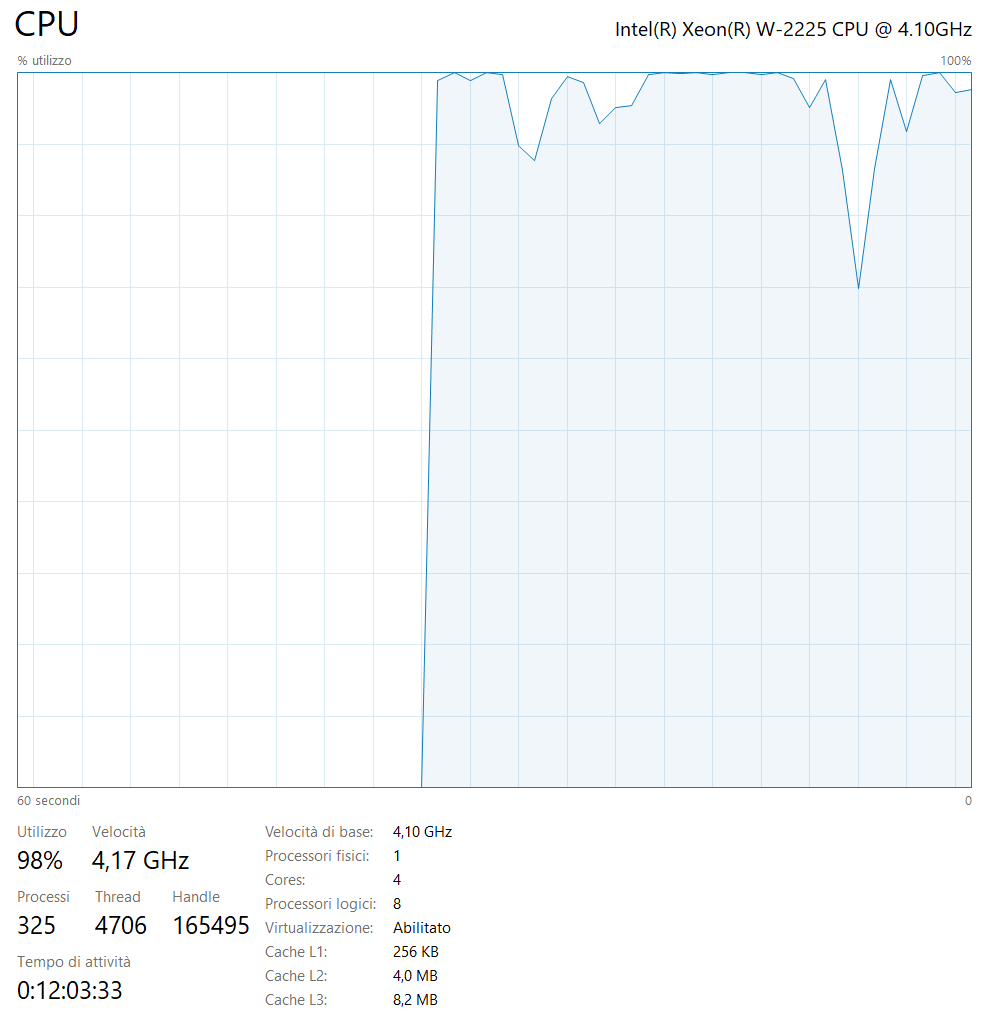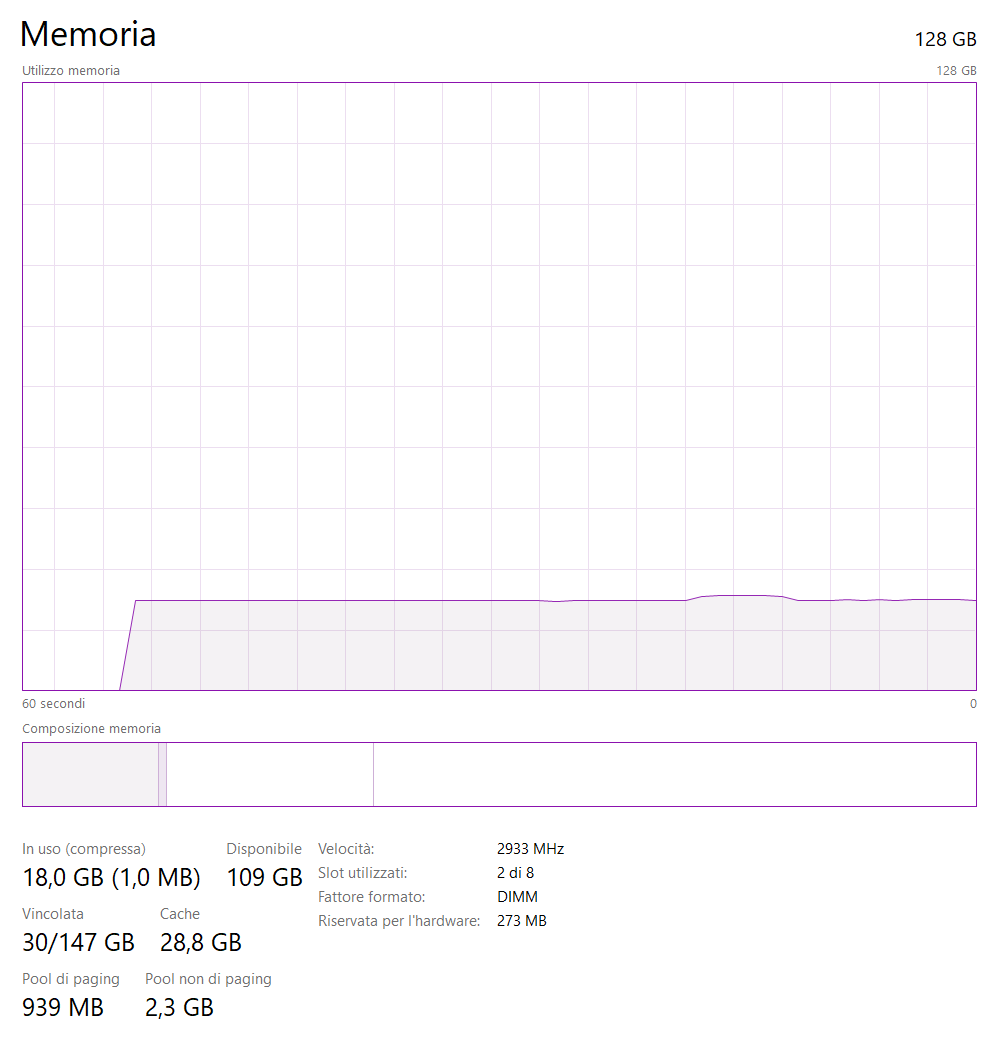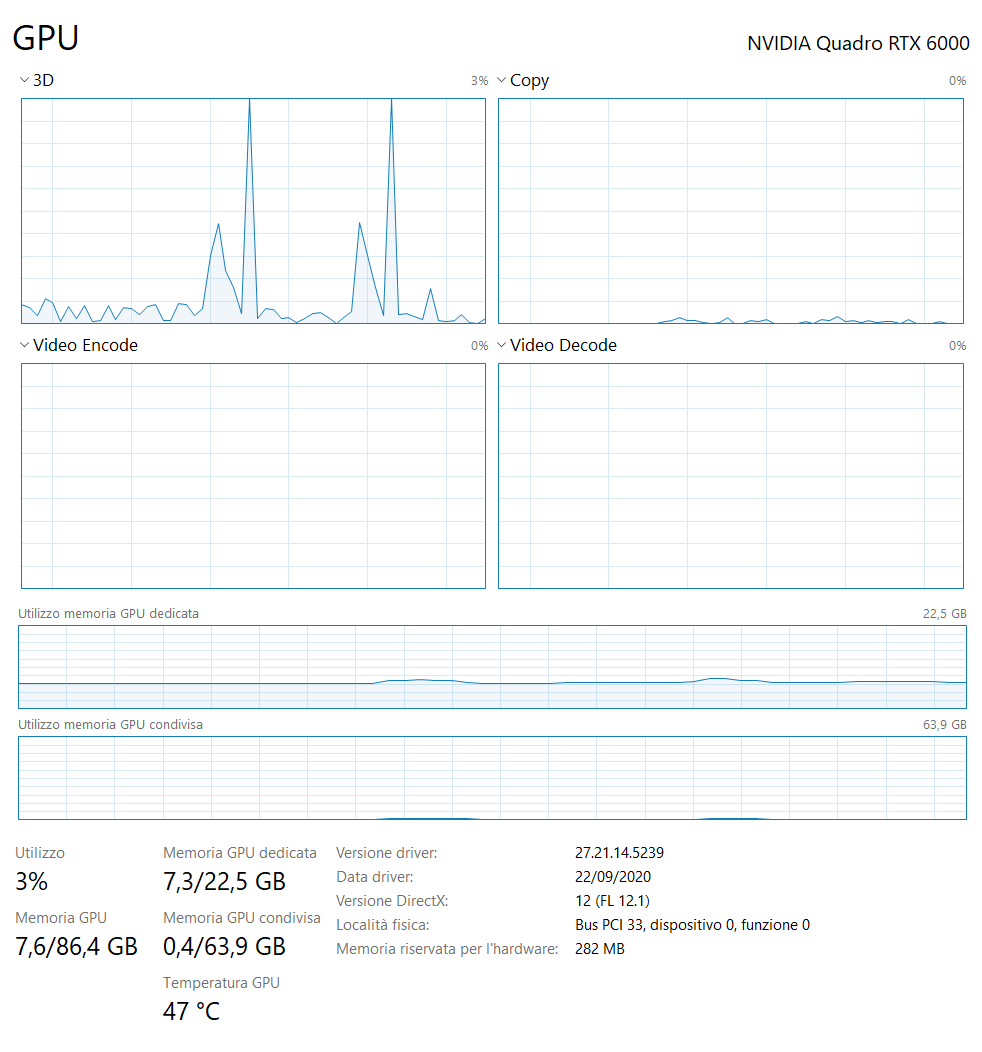This are the workstation counter during export phase

203 Messages

•

1.9K Points

As far as i have see there is new drivers released the 29/10/2020 for your video card. I will first install them

1.2K Messages

•

13.1K Points

1 y ago

Possible duplicate response, I accidentally killed the window while responding.

I'm doing everything you are on the prior generation HP hardware: Z440, 64GB RAM, Xeon 6-core 3.6/4.0...

Most likely cause is an incompatible plug-in. There is a flood of these problems reported with V10 upgrades.

It would be quite a coincidence but you could be experiencing a hardware failure, mostly likely with a SSD, suspect the one used for exports.

BTW - I'm jealous of your video card, I'm using a Quadro P2200 though it never goes above 35% busy, perhaps due to the 5GB of RAM.

1.2K Messages

•

13.1K Points

Everything looks normal to me. Here are the equivalents for my 300 RAW exported to JPEG test. What export settings are you using? All I did was choose sRGB at 100% quality and made no other changes to the images.

Still looks like a hardware failure to me, perhaps running out of space on the export drive. On my machine:

• C drive NVMe SSD - OS and software installs
• P drive - SATA SSD on PCIe bus - all Lightroom files and photos, nothing else on the drive
• W drive - SATA SSD on SATA port - export target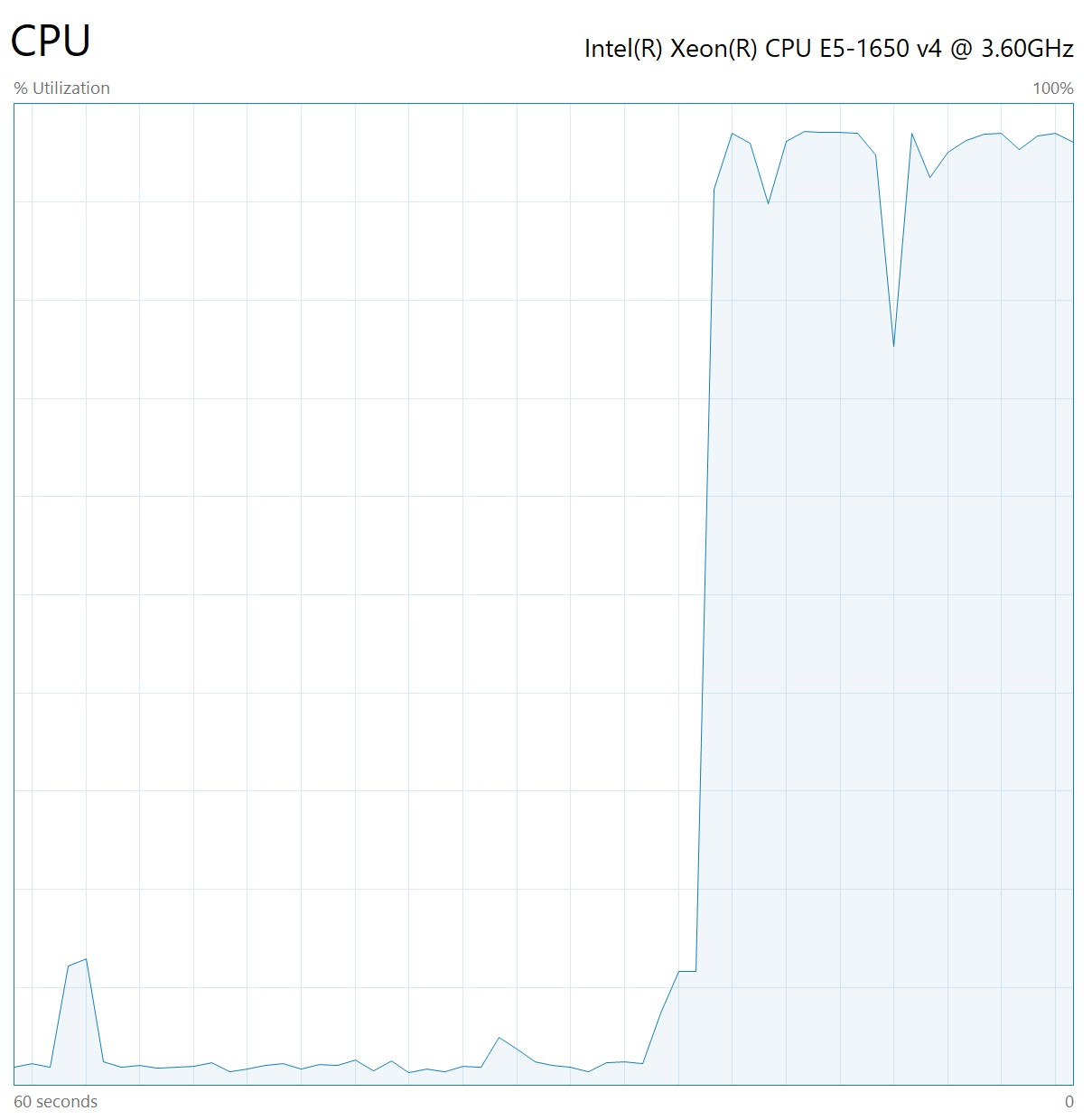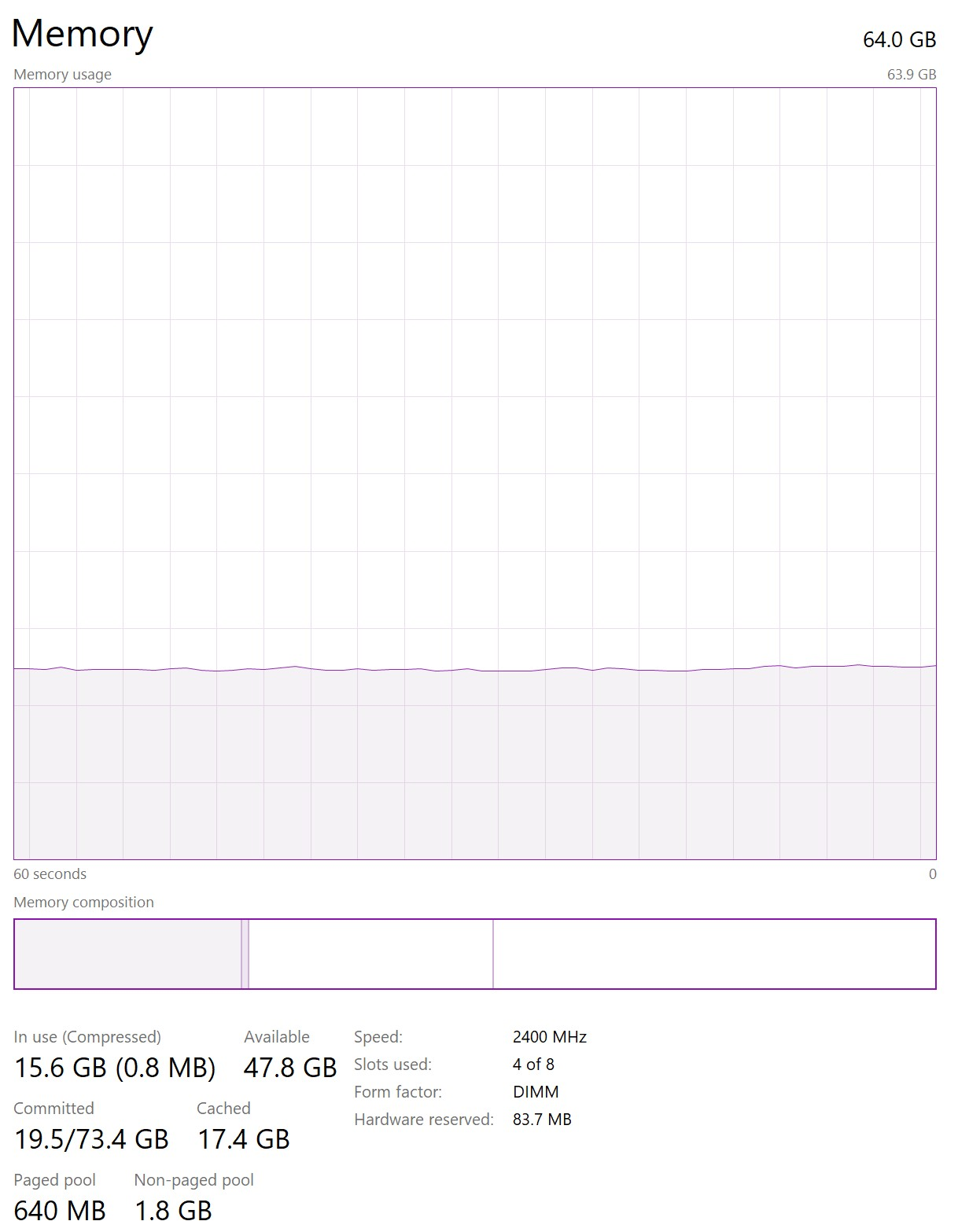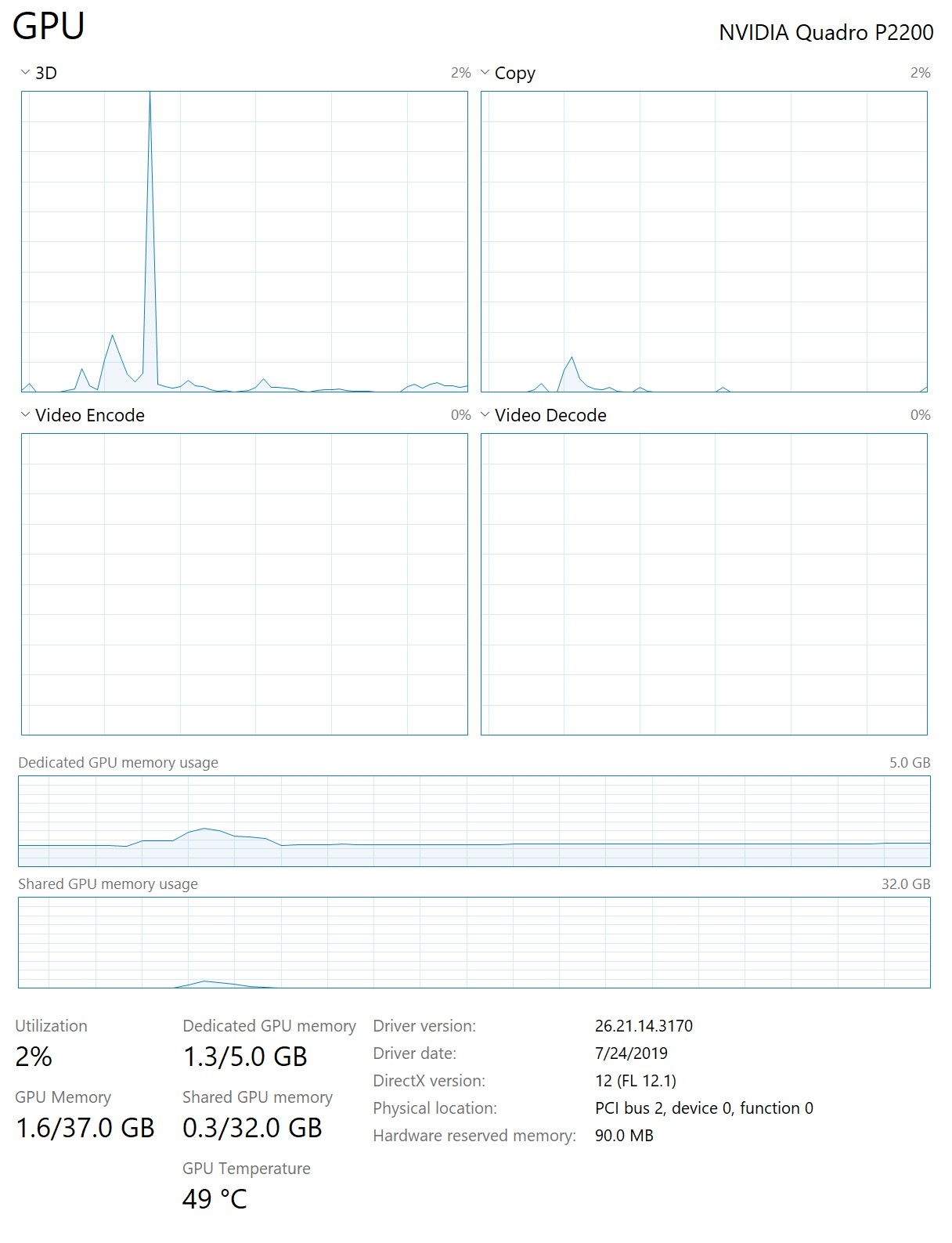8 Messages

•

140 Points

1 y ago

This happens on my mobile.

Especially when I deleted/moved a photo to another folder. Then when I try to export the same image, i get the error.

1.2K Messages

•

13.1K Points